×

×

/ What is the definition of generalize?

# What is the definition of generalize? Description

##### Description: Topics 4-7
6 Pages 37 Views 2 Unlocks
Reviews

Precalculus Algebra (MAC1140) Exam 2 Study Guide

## What is the definition of generalize?Topics 4-7

Topic 4: Extending to Models

- Definitions

o Generalize – a process of going from a (somewhat) specific problem to  a methodology or (Generalized) Model to attain answers to (less  specific) variations of the original problem.

o Variable – a symbol used to represent an unknown piece of data. o Independent variable – a variable whose value is unspecified but will  be provided by the statement of the problem.

o Dependent variable – a variable whose value is unknown and is  generated by the Model. In most cases dependent variables are  determined (solved for) by the Model.

o Arbitrary constant – a symbol that is unaffected by independent  variables, and whose value is determined by the model and initial  conditions of the system.

## What is the definition of an independent variable?We also discuss several other topics like What are some examples of autotrophs?

- Things that are repetitive are usually susceptible to being generalized. - Steps to Generalizing

o Determine what can be generalized

 Consider what information you need and already know what  (type of) units it would be supplied in.

o Determine what SHOULD be generalized and how

 Which of the things that I can generalize are likely to need to  change from project to project?

 If a piece of information is likely to change between different  variations of projects, then it’s a good candidate for

generalizing.

 You generalize a piece of information by replacing it in your

model with the correct type of variable

o Variables and their roles

 EX: building a floor

## What is the definition of a dependent variable?∙ Pieces of data

o The length and width of the (rectangular) floor Don't forget about the age old question of What are the fundamental components of an economic section?
If you want to learn more check out Who are the cultural (social) liberals?

o The area of the floor

o The cost of the tiles

o The size of a single tile

o The number of necessary tiles to make the floor We also discuss several other topics like What is the other term for manufacturing cost?

o The cost of labor to build the floor

o The time to build the floor

∙ Relationships

o The (number of tiles needed) is approximately the

(area of the floor) divided by the (area of the tiles)

o The (area of the tiles) is equal to the product of the

(width of the tiles) and the (length of the tiles)

o The (area of the floor) is the product of the (width

of the floor) and the (length of the floor)

o The (total cost of the tiles) is the product of the

(cost of a tile) and the (number of tiles needed)

o The (total cost of labor) is the product of the (hourly

cost of labor) and the (time to build the floor (in

hours))

o The (total cost of the floor) is the sum of the (total

cost of tiles) and the (total cost of labor)

∙ Create variables

oAF is the area of the floor (in square feet)

oAT is the area of a tile Don't forget about the age old question of What is the function of transport proteins?

oLF∧wF are the length and width of the floor

respectively

oLT∧wT are the length and width of the tiles

respectively

oCL is the cost of labor (in dollars)

oCT is the cost of the tiles (in dollars)

oCc is the total cost of the floor project (in dollars)

oT F is the total time to build the floor

∙ From there you can build relationships

oAF=LF×wF

Topic 5: Functions and their Roles in Models

- Definitions

o Mathematical relationship – a link between two or more pieces of

information or data.

o Mathematical expression – a statement that involves variables and/or  constants and some relationship between them. We also discuss several other topics like What is literature?

o Equation – mathematical expressions or relations that involve two or

more variables and an equality.

o Domain – valid input values for the independent variables of the

equation.

o Codomain – the set (or type) of values a dependent variable can

possibly have.

o Function – a specific type of mathematical relationship that relates

independent and dependent variables and yields precisely one value

for each dependent variable.

o Range – possible output values of the dependent variable of a given

function and domain pair.

- Example: Vending Machine

#1

#2

#3

#4

#5

A

Pepsi

Pepsi

Pepsi

Pepsi

Pepsi

B

Fanta

Fanta

Fanta

Fanta

Fanta

C

Dr. Pepper

Dr. Pepper

Dr. Pepper

Dr. Pepper

Dr. Pepper

D

Coke

Coke

Coke

Coke

Coke

E

Sprite

Sprite

Sprite

Sprite

Sprite

F

Root Beer

Root Beer

Root Beer

Root Beer

Root Beer

o Domain: A1-F5

o Co-Domain: soda

o Range: options in vending machine

o Function: input – letter, number; output – soda

o For any given combination of letter and number (Ex: B4) you know

exactly what drink you will get

o For a specific drink, you have multiple options

-R - real numbers

-Z - integers

- N - natural numbers

- Q - rational numbers

- Set builder notation

o For seats in a cafeteria

{s: s is a seat in this cafeteria}

o Seats in first 4 rows

{s:s is a seat in this cafeteria, s is in the front 4 rows}

{ x : x∈ R}

Topic 6: Graphing Functions

- Definitions

o Graph (of a function) – a visual representation of the relationship

between domain and range

o (cartesian) coordinate – a method of graphing a function where the

domain and range meet at a right angle

o Precision – how exact a number is.

 Ex: 0.54 is more precise than 0.54678

o Accuracy – how close to correct a value is.

 Ex: 2.2367 is more accurate than 2.567 to √5 (2.23606798)

- Information to look at when looking at a graph

o Continuity of the relationship: can be seen by seeing if there are any

breaks or jumps in the drawing of the graph

o Local and absolute extrema: maximum and minimum values of the

dependent variable

o Zeros: values of the independent variable that make the dependent

variable equal zero

o Steepness: we are used to thinking about slope in terms of linear  functions, but it turns out that you can determine how “steep” a  function is at a single point

Topic 7: Intro to the “Library of Functions”

- Definitions

o Parent function – the “prototypical” form of the given function type.  The most basic version of a function with no manipulations, shifts or  changes to its form.

- Polynomials

o Linear function

 Parent function: f(x) = x

 Graph: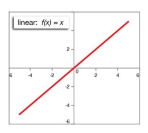 Notable features

∙ Only point of intersect is (0,0)

∙ Shape is a truly straight line

 Parent function: f(x) = x²

 Graph: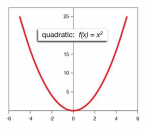 Notable features:

∙ Only point of intersect is (0,0) (vertex)

∙ Shape is called a parabola

o Cubic polynomials

 Parent function: f(x) = x3

 Graph: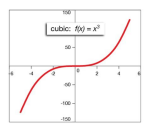 Notable features:

∙ Only point of intersect is (0,0) (vertex)

∙ Strictly increasing

o Parent function: f(x) = √ x

o Graph: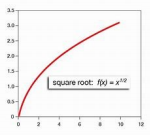o Notable features:

 point of special interest is (0,0) or (origin) of function  natural domain is only defined in the first quadrant - Absolute value

o Parent function: f(x) = |x|

o Graph: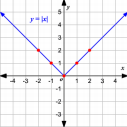o Notable features:

 (0,0) = vertex or reflection point

- Exponentials

o Parent function: f(x) = ⅇx

o Graph: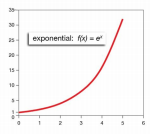o Notable features:

 (0,1) is a point of interest

 To left, graph approaches x-axis, but doesn’t touch it

- Logarithms

o Parent function: f(x) = ln ( x )

o Graph: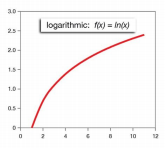o Notable features: (1,0) corresponds to (0,1) of exponential parent  function

Page ExpiredIt looks like your free minutes have expired! Lucky for you we have all the content you need, just sign up here